### Logistic Regressions with Random Intercepts

Researchers investigated the performance of two medical procedures in a multicenter study. They randomly selected 15 centers for inclusion. One of the study goals was to compare the occurrence of side effects for the procedures. In each center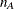patients were randomly selected and assigned to procedure A, and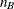patients were randomly assigned to procedure B. The following DATA step creates the data set for the analysis:

data multicenter;
input center group\$ n sideeffect;
datalines;
1  A  32  14
1  B  33  18
2  A  30   4
2  B  28   8
3  A  23  14
3  B  24   9
4  A  22   7
4  B  22  10
5  A  20   6
5  B  21  12
6  A  19   1
6  B  20   3
7  A  17   2
7  B  17   6
8  A  16   7
8  B  15   9
9  A  13   1
9  B  14   5
10  A  13   3
10  B  13   1
11  A  11   1
11  B  12   2
12  A  10   1
12  B   9   0
13  A   9   2
13  B   9   6
14  A   8   1
14  B   8   1
15  A   7   1
15  B   8   0
;


The variable group identifies the two procedures, n is the number of patients who received a given procedure in a particular center, and sideeffect is the number of patients who reported side effects.

If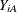and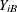denote the number of patients in center i who report side effects for procedures A and B, respectively, then—for a given center—these are independent binomial random variables. To model the probability of side effects for the two drugs,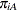and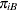, you need to account for the fixed group effect and the random selection of centers. One possibility is to assume a model that relates group and center effects linearly to the logit of the probabilities: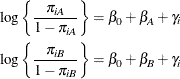In this model,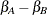measures the difference in the logits of experiencing side effects, and the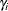are independent random variables due to the random selection of centers. If you think of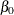as the overall intercept in the model, then theare random intercept adjustments. Observations from the same center receive the same adjustment, and these vary randomly from center to center with variance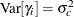.

Becauseis the conditional mean of the sample proportion,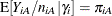, you can model the sample proportions as binomial ratios in a generalized linear mixed model. The following statements request this analysis under the assumption of normally distributed center effects with equal variance and a logit link function:

proc glimmix data=multicenter;
class center group;
model sideeffect/n = group / solution;
random intercept / subject=center;
run;


The PROC GLIMMIX statement invokes the procedure. The CLASS statement instructs the procedure to treat the variables center and group as classification variables. The MODEL statement specifies the response variable as a sample proportion by using the events/trials syntax. In terms of the previous formulas, sideeffect/n corresponds to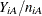for observations from group A and to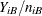for observations from group B. The SOLUTION option in the MODEL statement requests a listing of the solutions for the fixed-effects parameter estimates. Note that because of the events/trials syntax, the GLIMMIX procedure defaults to the binomial distribution, and that distribution’s default link is the logit link. The RANDOM statement specifies that the linear predictor contains an intercept term that randomly varies at the level of the center effect. In other words, a random intercept is drawn separately and independently for each center in the study.

The results of this analysis are shown in Figure 43.1Figure 43.9.

The Model Information Table in Figure 43.1 summarizes important information about the model you fit and about aspects of the estimation technique.

Figure 43.1: Model Information

The GLIMMIX Procedure

Model Information
Data Set WORK.MULTICENTER
Response Variable (Events) sideeffect
Response Variable (Trials) n
Response Distribution Binomial
Link Function Logit
Variance Function Default
Variance Matrix Blocked By center
Estimation Technique Residual PL
Degrees of Freedom Method Containment

PROC GLIMMIX recognizes the variables sideeffect and n as the numerator and denominator in the events/trials syntax, respectively. The distribution—conditional on the random center effects—is binomial. The marginal variance matrix is block-diagonal, and observations from the same center form the blocks. The default estimation technique in generalized linear mixed models is residual pseudo-likelihood with a subject-specific expansion (METHOD=RSPL).

The Class Level Information table lists the levels of the variables specified in the CLASS statement and the ordering of the levels. The Number of Observations table displays the number of observations read and used in the analysis (Figure 43.2).

Figure 43.2: Class Level Information and Number of Observations

Class Level Information
Class Levels Values
center 15 1 2 3 4 5 6 7 8 9 10 11 12 13 14 15
group 2 A B

 Number of Observations Read 30 30 155 503

There are two variables listed in the CLASS statement. The center variable has fifteen levels, and the group variable has two levels. Because the response is specified through the events/trial syntax, the Number of Observations table also contains the total number of events and trials used in the analysis.

The Dimensions table lists the size of relevant matrices (Figure 43.3).

Figure 43.3: Dimensions

Dimensions
G-side Cov. Parameters 1
Columns in X 3
Columns in Z per Subject 1
Subjects (Blocks in V) 15
Max Obs per Subject 2

There are three columns in thematrix, corresponding to an intercept and the two levels of the group variable. For each subject (center), thematrix contains only an intercept column.

The Optimization Information table provides information about the methods and size of the optimization problem (Figure 43.4).

Figure 43.4: Optimization Information

Optimization Information
Optimization Technique Dual Quasi-Newton
Parameters in Optimization 1
Lower Boundaries 1
Upper Boundaries 0
Fixed Effects Profiled
Starting From Data

The default optimization technique for generalized linear mixed models with binomial data is the quasi-Newton method. Because a residual likelihood technique is used to compute the objective function, only the covariance parameters participate in the optimization. A lower boundary constraint is placed on the variance component for the random center effect. The solution for this variance cannot be less than zero.

The Iteration History table displays information about the progress of the optimization process. After the initial optimization, the GLIMMIX procedure performed 15 updates before the convergence criterion was met (Figure 43.5). At convergence, the largest absolute value of the gradient was near zero. This indicates that the process stopped at an extremum of the objective function.

Figure 43.5: Iteration History and Convergence Status

Iteration History
Iteration Restarts Subiterations Objective
Function
Change Max
Gradient
0 0 5 79.688580269 0.11807224 7.851E-7
1 0 3 81.294622554 0.02558021 8.209E-7
2 0 2 81.438701534 0.00166079 4.061E-8
3 0 1 81.444083567 0.00006263 2.311E-8
4 0 1 81.444265216 0.00000421 0.000025
5 0 1 81.444277364 0.00000383 0.000023
6 0 1 81.444266322 0.00000348 0.000021
7 0 1 81.44427636 0.00000316 0.000019
8 0 1 81.444267235 0.00000287 0.000017
9 0 1 81.444275529 0.00000261 0.000016
10 0 1 81.44426799 0.00000237 0.000014
11 0 1 81.444274843 0.00000216 0.000013
12 0 1 81.444268614 0.00000196 0.000012
13 0 1 81.444274277 0.00000178 0.000011
14 0 1 81.444269129 0.00000162 9.772E-6
15 0 0 81.444273808 0.00000000 9.102E-6

 Convergence criterion (PCONV=1.11022E-8) satisfied.

The Fit Statistics table lists information about the fitted model (Figure 43.6).

Figure 43.6: Fit Statistics

Fit Statistics
-2 Res Log Pseudo-Likelihood 81.44
Generalized Chi-Square 30.69
Gener. Chi-Square / DF 1.10

Twice the negative of the residual log likelihood in the final pseudo-model equaled 81.44. The ratio of the generalized chi-square statistic and its degrees of freedom is close to 1. This is a measure of the residual variability in the marginal distribution of the data.

The Covariance Parameter Estimates table displays estimates and asymptotic estimated standard errors for all covariance parameters (Figure 43.7).

Figure 43.7: Covariance Parameter Estimates

Covariance Parameter Estimates
Cov Parm Subject Estimate Standard Error
Intercept center 0.6176 0.3181

The variance of the random center intercepts on the logit scale is estimated as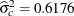.

The Parameter Estimates table displays the solutions for the fixed effects in the model (Figure 43.8).

Figure 43.8: Parameter Estimates

Solutions for Fixed Effects
Effect group Estimate Standard Error DF t Value Pr > |t|
Intercept   -0.8071 0.2514 14 -3.21 0.0063
group A -0.4896 0.2034 14 -2.41 0.0305
group B 0 . . . .

Because of the fixed-effects parameterization used in the GLIMMIX procedure, the Intercept effect is an estimate of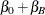, and the A group effect is an estimate of, the log odds ratio. The associated estimated probabilities of side effects in the two groups are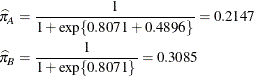There is a significant difference between the two groups (p = 0.0305).

The Type III Tests of Fixed Effect table displays significance tests for the fixed effects in the model (Figure 43.9).

Figure 43.9: Type III Tests of Fixed Effects

Type III Tests of Fixed Effects
Effect Num DF Den DF F Value Pr > F
group 1 14 5.79 0.0305

Because the group effect has only two levels, the p-value for the effect is the same as in the Parameter Estimates table, and the F Value is the square of the t Value shown there.

You can produce the estimates of the average logits in the two groups and their predictions on the scale of the data with the LSMEANS statement in PROC GLIMMIX:

ods select lsmeans;
proc glimmix data=multicenter;
class center group;
model sideeffect/n = group / solution;
random intercept / subject=center;
lsmeans group / cl ilink;
run;


The LSMEANS statement requests the least squares means of the group effect on the logit scale. The CL option requests their confidence limits. The ILINK option adds estimates, standard errors, and confidence limits on the mean (probability) scale (Figure 43.10).

Figure 43.10: Least Squares Means

The GLIMMIX Procedure

group Least Squares Means
group Estimate Standard Error DF t Value Pr > |t| Alpha Lower Upper Mean Standard
Error
Mean
Lower
Mean
Upper
Mean
A -1.2966 0.2601 14 -4.99 0.0002 0.05 -1.8544 -0.7388 0.2147 0.04385 0.1354 0.3233
B -0.8071 0.2514 14 -3.21 0.0063 0.05 -1.3462 -0.2679 0.3085 0.05363 0.2065 0.4334

The Estimate column displays the least squares mean estimate on the logit scale, and the Mean column represents its mapping onto the probability scale. The Lower and Upper columns are 95% confidence limits for the logits in the two groups. The Lower Mean and Upper Mean columns are the corresponding confidence limits for the probabilities of side effects. These limits are obtained by inversely linking the confidence bounds on the linear scale, and thus are not symmetric about the estimate of the probabilities.